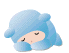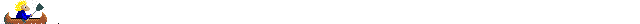~Conjectures and Counterexamples

~CONJECTURES.... and....COUNTEREXAMPLES~

CONJECTURE
is a conclusion based on examples.
COUNTEREXAMPLE
is an example that shows a conjecture is to be false.

Example of Conjectures for a rectangle

a) If a quadrilateral has four equal angles, it has four equal sides.
b) The length of a rectangle is always double its width
c) The opposite sides of a parallelogram are equal in length.

Out of the three conjectures given, a) and b) are the counterexamples.Examples of false conjecture with their true counterexamples

A number that is not positive is negative.
0
If 1 is added to an off number, the result is always an even number
1 squared=1
The altitude of a triangle always lies inside the triangle
An altitude of an obtuse triangle lies outside it.
Every rectangle is a square
Any rectangle in which its length is not equal to its width is not a square.